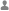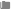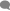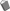## array_merge与函数运算符“+”加号的区别Veris分类：PHP0条评论已被围观 802
1. array_merge在参考手册中的说明如下：
array_merge()将两个或多个数组的单元合并起来，一个数组中的值附加在前一个数组的后面。返回作为结果的数组。

2. 对于使用“＋”合并数组：

```<?php
\$a1 = array(1 => "first", "2" => "second", 3 => "third");
\$a2 = array(2 => "second value", 3 => "3rd");
print_r(\$a1 + \$a2);
print_r(array_merge(\$a1, \$a2));```

```Array
(
 => first
 => second
 => third
)
Array
(
 => first
 => second
 => third
 => second value
 => 3rd
)```﻿